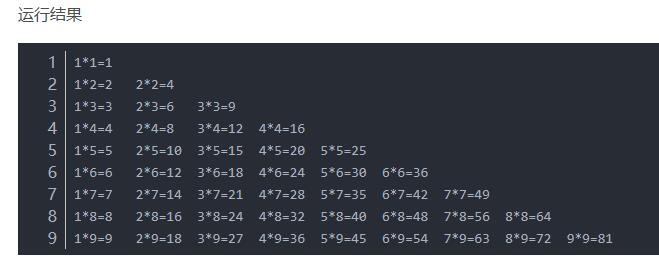2020-06-13 15:53

# 高分悬赏：Java语言怎么输出一个9x9的乘法口诀表，输出的结果要三角形的怎么实现

• 点赞
• 写回答
• 关注问题
• 收藏
• 邀请回答

#### 5条回答默认 最新

•毕小宝 2020-06-30 08:29
已采纳

楼主请看这里：https://blog.csdn.net/fox_triumph/article/details/88853189点赞 评论
•梁聪俊 2020-06-13 16:39

package coom;

public class MultiplicationTable {
public static void main(String[] args) {
for (int i = 0; i < 10; ++i) {
System.out.println();
for (int j = 1; j < i + 1; j++) {
System.out.printf("%2d*%2d=%d ", j, i, (i * j));
}
}
}
}

点赞 评论
•南极墨白 2020-06-13 17:19

## 代码如下

``````public class Main {
public static void main(String[] args) {
for (int i = 1; i < 10; i++) {
for (int j = 1; j <= i; j++) {
System.out.printf("%dx%d=%d\t", i, j, i * j);  //用一个tab 隔开每个式子
}
System.out.println();  //换行
}
}
}
``````

## 结果如下

``````1x1=1
2x1=2   2x2=4
3x1=3   3x2=6   3x3=9
4x1=4   4x2=8   4x3=12  4x4=16
5x1=5   5x2=10  5x3=15  5x4=20  5x5=25
6x1=6   6x2=12  6x3=18  6x4=24  6x5=30  6x6=36
7x1=7   7x2=14  7x3=21  7x4=28  7x5=35  7x6=42  7x7=49
8x1=8   8x2=16  8x3=24  8x4=32  8x5=40  8x6=48  8x7=56  8x8=64
9x1=9   9x2=18  9x3=27  9x4=36  9x5=45  9x6=54  9x7=63  9x8=72  9x9=81
``````
点赞 评论
•爆汁榴莲 2020-06-13 20:22

public class 乘法表 {

``````public static void main(String[] args) {

for (int n=1; n<=9; n++) {                               // i是一个乘数
for (int m=1; m<=n; m++) {                    // j是另一个乘数
System.out.print(m+"*"+n+"="+(m*n)+"\t");
}
System.out.println();
}
}
}
``````
``````这是嵌套循环的内容  希望能接纳
``````

结果：
1*1=1

1*2=2 2*2=4

1*3=3 2*3=6 3*3=9

1*4=4 2*4=8 3*4=12 4*4=16

1*5=5 2*5=10 3*5=15 4*5=20 5*5=25

1*6=6 2*6=12 3*6=18 4*6=24 5*6=30 6*6=36

1*7=7 2*7=14 3*7=21 4*7=28 5*7=35 6*7=42 7*7=49

1*8=8 2*8=16 3*8=24 4*8=32 5*8=40 6*8=48 7*8=56 8*8=64

1*9=9 2*9=18 3*9=27 4*9=36 5*9=45 6*9=54 7*9=63 8*9=72 9*9=81

点赞 评论
•qq_26868981 2020-06-17 08:47

你不会，，，一个简单地的hello world都没运行出来吧，这个关键不就是两个循环那一复制拷贝到主函数就行了，还有什么运行不了的。要是连hello world都没运行出来，那就真没办法了

点赞 评论